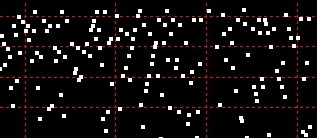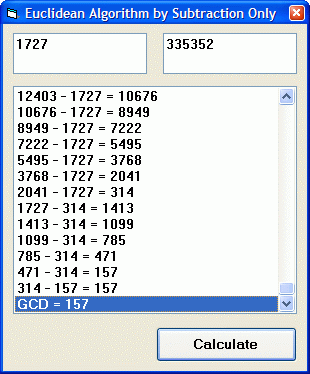exploring the undesigned
intelligence of the numberversehome

 Euclidean Algorithm Using Subtraction Only Explanations of the Euclidean Algorithm for finding the greatest common divisor of two integers often seem long and convoluted. The idea is, in fact, beautifully simple. All it is is a process of repeat subtraction, carrying the result forward each time until the result is equal to the amount being subtracted. If the answer is greater than 1, there is a GCD (besides 1). If the answer is 1, there is no common divisor (besides 1), and so both numbers are coprime, or relative prime, to each other. The subtractive algorithm is simply this, where you 'rinse and repeat' the inner loops as long as a is not equal to b.  Do While a <> b      Do While a > b          c = a – b          a = c      Loop      Do While b > a          c = b – a          b = c      Loop  Loop What you're left with is a or b equals 1, no GCD, or a or b is greater than 1, a GCD. Perhaps the simplicity of the algorithm is obscured by the usual practice of using modular division. In fact, modular division is not required - and the Greeks certainly did not use it! However, the Euclidean algorithm is also a great demonstration of the power of modular arithmetic. Test the following VBA/VB6 example with, say, a 10-digit number and a 3-digit number. The subtraction method will take thousands of iterations to produce a result. (If you get an overflow, it's probably because you've exceeded the listbox limit.) Then try replacing subtraction with the couple of lines of modular division in the code below, and see how the result is achieved within just a few iterations and a fraction of a second.VBA/VB6 Source Code Requires a form with two text boxes, a listbox, and a command button. Option Explicit Private Sub Command1_Click() Dim a As Variant, b As Variant, c As Variant List1.Clear If Val(Text1) > Val(Text2) Then a = CDec(Val(Text1)) b = CDec(Val(Text2)) Else a = CDec(Val(Text2)) b = CDec(Val(Text1)) End If If a = 0 Or b = 0 Then Exit Sub Repeat: Do While a > b c = a - b 'remove for modular '''''''''For Modular Division''''''''''''' ' If b = 0 Then GoTo Finish ' c = a Mod b '''''''''''''''''''''''''''''''''''''''''' List1.AddItem Trim\$(a) + " - " + Trim\$(b) + " = " + Trim\$(c) List1.ListIndex = List1.ListCount - 1 a = c Me.Refresh DoEvents Loop Do While b > a c = b - a 'remove for modular '''''''''For Modular Division''''''''''''' ' If a = 0 Then GoTo Finish ' c = b Mod a '''''''''''''''''''''''''''''''''''''''''' List1.AddItem Trim\$(b) + " - " + Trim\$(a) + " = " + Trim\$(c) List1.ListIndex = List1.ListCount - 1 b = c Me.Refresh DoEvents Loop If a <> b Then GoTo Repeat '''''''''For Modular Division''''''''''''' 'Finish: ' List1.AddItem "GCD =" + Str\$(b) '''''''''''''''''''''''''''''''''''''''''' List1.AddItem "GCD =" + Str\$(a) 'remove for modular! List1.Selected(List1.ListCount - 1) = True End Sub   © 2008 Michael M. Ross